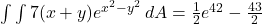## 7(x + y) ex2 − y2 dA, R where R is the rectangle enclosed by the lines x − y = 0, x − y = 7, x + y = 0, and x + y = 6

Question

7(x + y) ex2 − y2 dA, R where R is the rectangle enclosed by the lines x − y = 0, x − y = 7, x + y = 0, and x + y = 6

in progress 0
2 months 2021-08-31T14:01:11+00:00 1 Answers 0 views 0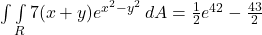Step-by-step explanation:

Given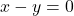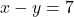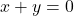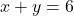Required

Evaluate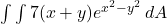Let: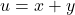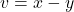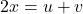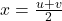Subtract both equations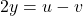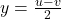So:R is defined by the following boundaries: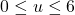,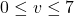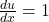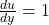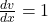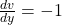So, we can not set up Jacobian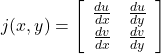This gives: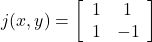Calculate the determinant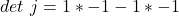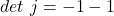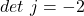Now the integral can be evaluated:becomes: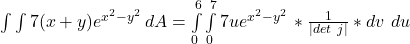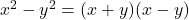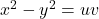So: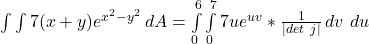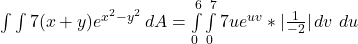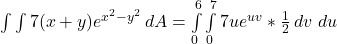Remove constants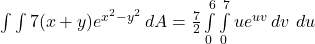Integrate v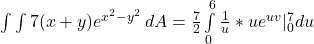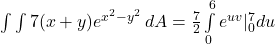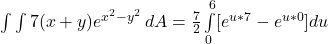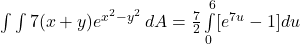Integrate u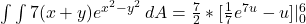Expand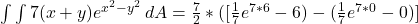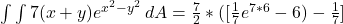Open bracket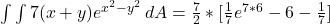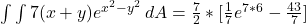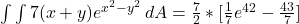Expand